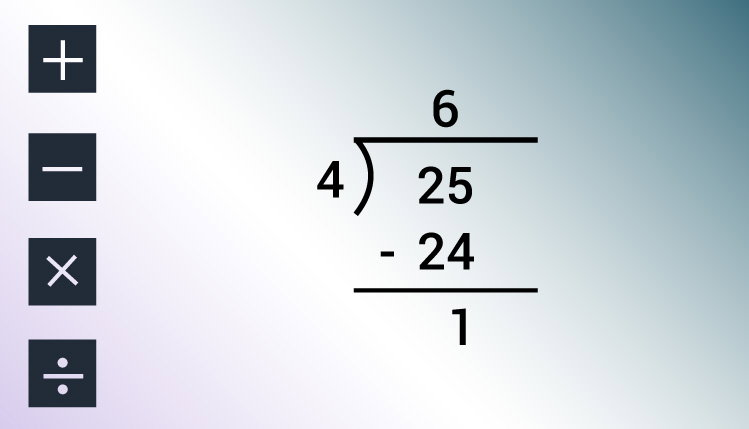# C Program to Compute Quotient and Remainder

This program evaluates the quotient and remainder when an integer is divided by another integer.To understand this example, you should have the knowledge of following C programming topics:

## Program to Compute Quotient and Remainder

``````#include <stdio.h>
int main(){

int dividend, divisor, quotient, remainder;

printf("Enter dividend: ");
scanf("%d", &dividend);

printf("Enter divisor: ");
scanf("%d", &divisor);

// Computes quotient
quotient = dividend / divisor;

// Computes remainder
remainder = dividend % divisor;

printf("Quotient = %d\n", quotient);
printf("Remainder = %d", remainder);

return 0;
}
``````

Output

```Enter dividend: 25
Enter divisor: 4
Quotient = 6
Remainder = 1```

In this program, user is asked to enter two integers (dividend and divisor) which is stored in variable dividend and divisor respectively.

Then the quotient is evaluated using division / operator and stored in variable quotient.

Similarly, the remainder is evaluated using modulus % operator and stored in remainder variable.

Finally, the quotient and remainder are displayed using `printf()` function.